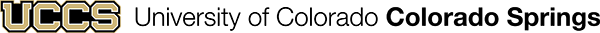# Mathematics Research: Outlines, Practice Problems & Alternative Textbooks

This guide has information about where to find math books and study guides on various topics, a tool that lets you search for your textbooks in the library, and links to databases where you can search for math articles.

Math books are in the QA section of the Library's Main Collection. (There are also many math ebooks.) This is a large section so the boxes below provide information on where to browse for books in specific areas of mathematics.

## Schaum's Outlines and Solved Problems

Schaum's Outlines provide overviews of a topic and include hundreds of solved problems that you can use to practice your skills.

## Abstract Algebra

QA162 (QA174.2 for Group Theory)

Some examples of books in that section:

## College Algebra / Precalculus

QA152-154

Some examples of books in that section:

## Linear Algebra

QA184 OR QA251

Some examples of books in those sections:

## Analysis

QA300 OR QA331 (Real Analysis)

Some examples of books in those sections.

## Differential Equations

QA370-372 (Ordinary Differential Equations)

QA374-379 (Partial Differential Equations)

Some examples of books in those sections:

## Probability

QA273

Some examples of books in this section:

## Calculus

QA303

Some examples of books in that section:

## Statistics

QA276

Some examples of books in this section: